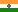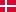## New Drugs and Clinical Trials Rules: Wrong definition of BE [GxP / QC / QA]

Dear Ohlbe and all,

» I could not find a definition of an "impartial witness" in the New Drugs and Clinical Trials Rules, 2019.

Thank you for pointing me to this reference! Unfortunately BE is wrongly defined (Chapter I, 2. Definitions (f), page 148):

“bioequivalence study” means a study to establish the absence of a statistically significant difference in the rate and extent of absorption of an active ingredient from a pharmaceutical formulation in comparison to the reference formulation having the same active ingredient when administered in the same molar dose under similar conditions;

(my emphases)

One error and a doubtful term:
1. Statistically significant? No way. Maybe the CDSCO’s gurus had a look at the FDA’s definition given in the CFR21 I D §320.23 (b)(1):
• Two drug products will be considered bioequivalent drug products if they are pharmaceutical equivalents or pharmaceutical alternatives whose rate and extent of absorption do not show a significant difference when administered at the same molar dose of the active moiety under similar experimental conditions, either single dose or multiple dose.
Note the absence of ‘statistically’. In the FDA’s definition ‘significant’ is used in its common meaning (1 or 2a). If BE would require “absence of a statistically significant difference”,should consider a union with.*
2. Similar conditions? Nope. They should be the same (food, beverages, time of administration, physical activity,). Though similar is also stated in the FDA’s definition, it is not mentioned in the EMA’s BE-GL (common sense!)…
This reminds me on a story Salomon Stavchansky once told me. He wrote more or less single-handed ANVISA’s first guidances, only to discover that a wrong definition of bioavailability was not only stated in the guidance (Resolução) but also in the law (Legislação). Whilst the former could have been corrected rather quickly, it took Brazil two years to change the latter.

• Example for a drug with low variability. Minimum sample size (in India only if justified 12), 14 subjects dosed, no dropouts; consequently extremely high power:
library(PowerTOST) CV  <- 0.1 n   <- 14 pe  <- seq(0.84, 1, length.out=100) pe  <- sort(unique(c(pe, 1/pe))) res <- data.frame(pe=100*pe, lower=NA, upper=NA, BE=FALSE, PE=FALSE, p=NA) for (j in seq_along(pe)) {   res[j, 2:3] <- round(100*CI.BE(pe=pe[j], CV=CV, n=n), 2)   if (res$lower[j] >= 80 & res$upper[j] <= 125) res$BE[j] <- TRUE if (res$lower[j] < 100 & res$upper[j] > 100) res$PE[j] <- TRUE   res$p[j] <- pvalue.TOST(pe=pe[j], CV=CV, n=n) } op <- par(no.readonly=TRUE) par(pty="s") plot(pe, res$p, type="n", log="x", xlab="point estimate", ylab="p", las=1) grid(); abline(h=0.05) box() lines(pe, res$p, lwd=3, col="red") lines(res$pe[res$BE == TRUE]/100, res$p[res$BE == TRUE], lwd=3, col="magenta") lines(res$pe[res$PE == TRUE]/100, res$p[res\$PE == TRUE], lwd=3, col="blue") legend("top", inset=0.02, box.lty=0, bg="white", lwd=3,        col=c("red", "magenta", "blue"),        legend=c("fails BE", "passes BE", "n.s. (CI includes 1)")) par(op)Studies with point estimates of 85.6–116.8% pass everywhere except in, where the PE has to lie within 93.5–106.9%. Bizarre.

Dif-tor heh smusma 🖖Helmut SchützThe quality of responses received is directly proportional to the quality of the question asked. 🚮
Science QuotesIng. Helmut Schütz